JEE  >  BITSAT Maths Test - 4

# BITSAT Maths Test - 4

Test Description

## 45 Questions MCQ Test BITSAT Mock Tests Series & Past Year Papers | BITSAT Maths Test - 4

BITSAT Maths Test - 4 for JEE 2023 is part of BITSAT Mock Tests Series & Past Year Papers preparation. The BITSAT Maths Test - 4 questions and answers have been prepared according to the JEE exam syllabus.The BITSAT Maths Test - 4 MCQs are made for JEE 2023 Exam. Find important definitions, questions, notes, meanings, examples, exercises, MCQs and online tests for BITSAT Maths Test - 4 below.
Solutions of BITSAT Maths Test - 4 questions in English are available as part of our BITSAT Mock Tests Series & Past Year Papers for JEE & BITSAT Maths Test - 4 solutions in Hindi for BITSAT Mock Tests Series & Past Year Papers course. Download more important topics, notes, lectures and mock test series for JEE Exam by signing up for free. Attempt BITSAT Maths Test - 4 | 45 questions in 60 minutes | Mock test for JEE preparation | Free important questions MCQ to study BITSAT Mock Tests Series & Past Year Papers for JEE Exam | Download free PDF with solutions
 1 Crore+ students have signed up on EduRev. Have you?
BITSAT Maths Test - 4 - Question 1

### Locus of the point, the sum of squares of whose distances from the points (a,0) and (-a,0) is equal to 2c2 is

BITSAT Maths Test - 4 - Question 2

### If T₂/T₃ in the expansion of (a+b)n and T₃/T₄ in the expansion of (a+b)(n+3) are equal, then n=

BITSAT Maths Test - 4 - Question 3

### The locus of mid point of the chords of the circle x2+y2-2x-6y-10=0 passing through the origin is

BITSAT Maths Test - 4 - Question 4

Let z₁ and z₂ be two roots of the equation z2 + az + b = 0,z being complex . Further ,assume that the origin ,z₁ and z₂ form an equilateral triangle .Then

BITSAT Maths Test - 4 - Question 5

The solution of the equation (dy/dx)=cotx coty is

Detailed Solution for BITSAT Maths Test - 4 - Question 5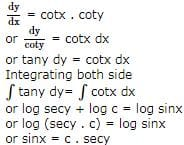BITSAT Maths Test - 4 - Question 6

AOB is the positive quadrant of the ellipse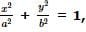, where OA = a, OB = b. The area between the arc AB and the chord AB of the ellipse is

Detailed Solution for BITSAT Maths Test - 4 - Question 6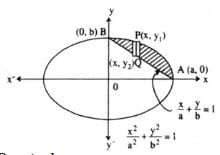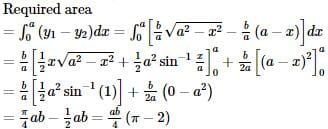BITSAT Maths Test - 4 - Question 7

If y=a cos (log x) + b sin (log x) where a,b are parametres, then x2y+xy'

Detailed Solution for BITSAT Maths Test - 4 - Question 7

Explanation : y = acos(logx) + bsin(logx)

dy/dx = -asin(logx)/x + bsin(logx)/x

xdy/dx = -asin(logx) + bsin(logx)

xd2y/dx2 + dy/dx = -acos(logx)/x - bsin(logx)/x

x2d2y/dx2 + xdy/dx = -acos(logx) - bsin(logx)

x2d2y/dx2 + xdy/dx = -y

BITSAT Maths Test - 4 - Question 8

The general solution of the equation x2dy=-2xydx is

BITSAT Maths Test - 4 - Question 9

If f(x)=logx2log(x), then at x=e, f'(x)=

BITSAT Maths Test - 4 - Question 10

The function f(x)=x3-3x2-24x+5 is increasing in the interval

BITSAT Maths Test - 4 - Question 11

1/(1.2.3.4) + 4/(3.4.5.6) + 9/(5.6.7.8) + 16/(7.8.9.10) + ....... ∞ =

BITSAT Maths Test - 4 - Question 12

The angle of elevation of the top of a tower from the top and bottom of a building of height 'a' are 30o and 45o respectively. If the tower and the building stand at the same level, the height of the tower is

BITSAT Maths Test - 4 - Question 13

The curve represented by x = a (cosh θ + sinh θ), y = b (cosh θ − sinh θ)    is

BITSAT Maths Test - 4 - Question 14

The parabolas y2 = 4x and x2 = 4y divide the square region bounded by the lines x = 4, y = 4 and the coordinate axes. If S₁, S₂, S₃ are respectively the areas of these parts numbered from top to bottom; then S₁ : S₂ : S₃ is

BITSAT Maths Test - 4 - Question 15

The area of the region bounded by the curves y = |x-1| and y = 3-|x| is

BITSAT Maths Test - 4 - Question 16

If cos-1p + cos-1q+cos-1r = π, then p2 + q2 + r2 + 2pqr is equal to

Detailed Solution for BITSAT Maths Test - 4 - Question 16

Let cos-1 p = α, cos-1 q = β and cos-1 r = γ
⇒ cosα = p, cosβ = q and cosγ = r
From question, α + β + γ = π
∴ cos (α + β) = cos (π - γ)
or, cosα cosβ - sinα sinβ = - cosγ
or pq - 1 − p 2 1 − q 2 = − r
or, pq + r = 1 − p 2 1 − q 2
Squaring, we get p2q2 + r2 + 2pqr = 1 - p2 - q2 + p2q2
or, p2 + q2 + r2 + 2pqr = 1

BITSAT Maths Test - 4 - Question 17

The value of α for which the function f(x)=1+αx, α≠0 is the inverse of itself, is

BITSAT Maths Test - 4 - Question 18

The difference of an integer and its cube is divisible by

BITSAT Maths Test - 4 - Question 19

x₁+2x₂+3x₃ = 2x₁+3x₂+x₃ = 3x₁+x₂+2x₃ = 0. This system of equation has

BITSAT Maths Test - 4 - Question 20

If the traces of the matrices A and B are 20 and -8, then the trace of (A + B)=

BITSAT Maths Test - 4 - Question 21

A cylindrical gas container is closed at the top and open at the bottom. If the iron plate of the top is 5/4 times as thick as the plate forming the cylindrical sides, the ratio of the radius to the height of the cylinder using minimum material for the same capacity is

BITSAT Maths Test - 4 - Question 22

(sin θ + i cos θ) 4

BITSAT Maths Test - 4 - Question 23

Lines x2+4xy+y2=0 and x-y=4 form the figure

BITSAT Maths Test - 4 - Question 24

Equation of the tangent at (-4,-4) on x2=-4y is

BITSAT Maths Test - 4 - Question 25

On the parabola y2 = 8 x if one extremity of a focal chord is (1 ∕ 2, − 2) then its other extremity is

BITSAT Maths Test - 4 - Question 26

How many words can be formed from the letters of the word COMMITTEE?

BITSAT Maths Test - 4 - Question 27

The numbers of all words formed from the letters of the word CALCUTTA is

Detailed Solution for BITSAT Maths Test - 4 - Question 27

No of letter in the given word is 8 No of repeated word is "C" 2 times, "A" 2 times and "T" 2 times. So the equation is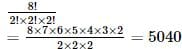BITSAT Maths Test - 4 - Question 28

Out of 30 consecutive integers, 2 are chosen at random. The probability that their sum is odd, is

BITSAT Maths Test - 4 - Question 29

Three identical dice are rolled. The probability that the same number will appear on each of them is

Detailed Solution for BITSAT Maths Test - 4 - Question 29

Total number of ways = 6 × 6 × 6 = 63 Number of favourable ways = 6 ∴ probability of the required event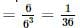BITSAT Maths Test - 4 - Question 30

If two angles of a Δ A B C are 45o and 600then the ratio of the smallest and the greatest sides are

BITSAT Maths Test - 4 - Question 31

In a triangle the line joining circum-centre and in-centre is parallel to BC, then cosB+cosC=

BITSAT Maths Test - 4 - Question 32

The value of p for which the difference between the roots of the equation x2+px+8 = 0 is 2, are

BITSAT Maths Test - 4 - Question 33

If a, b, c be in G.P. and p, q be respectively A.M., between a, b and b, c then

BITSAT Maths Test - 4 - Question 34

The sum of first n terms of the series (3/12) + (5/(12 + 22)) + (7/(12 + 22 + 32)) + ... is

BITSAT Maths Test - 4 - Question 35

If f (x) = (x)/(x - 1) = 1/y, then f (y) =

BITSAT Maths Test - 4 - Question 36

If f (x) = x/logx then 2f(x - 2) > f(x) if

BITSAT Maths Test - 4 - Question 37

If lines 3y+4x=1, y=x+5 and 5y+bx=3 are concurrent, then the value of b is

BITSAT Maths Test - 4 - Question 38

The length of the sub normal to the parabola y2=4ax at any point is equal to

BITSAT Maths Test - 4 - Question 39

The smallest radius of the sphere passing thro'(1,0,0),(0,1,0) and (0,0,1) is

Detailed Solution for BITSAT Maths Test - 4 - Question 39

Let the sphere be
x2 + y2 + z2 + 2ux + 2vy + 2wz + d' = 0 ...(i)
It passes through the points (1, 0, 0), (0, 1, 0), (0, 0, 1)
∴ 1 + 2y + d' = 0...(ii)
1 + 2v + d' = 0 ...(iii)
1 + 2w + d' = 0 ...(iv)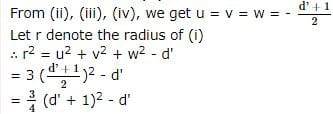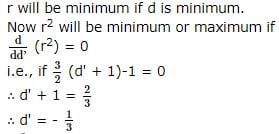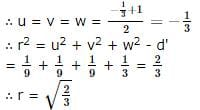BITSAT Maths Test - 4 - Question 40

The d.r. of normal to the plane through (1,0,0), (0,1,0) which makes an angle π/4 with plane x + y = 3 are

BITSAT Maths Test - 4 - Question 41

The general solution of the equation tan2θ.tanθ=1 for n∈I is, θ is equal to

Detailed Solution for BITSAT Maths Test - 4 - Question 41 Concept: General solutiontan tan2x.tan x = 1. tan x = 1/(tan 2x) =cot 2x. tan x = tan (π/2 -2x). (general solution of tan x = nπ+x.) x = nπ +(π/2–2x). x+2x= (n+1/2)π 3x = (2n+1)π/2. x = (2n+1)π/6. Hence, correct answer is (B).
BITSAT Maths Test - 4 - Question 42

If sinθ=√3cosθ, -π<θ<0, θ=

BITSAT Maths Test - 4 - Question 43

cos 255o + sin 165o=

BITSAT Maths Test - 4 - Question 44

If p=7i-2j+3k and q=3i+j+5k, then the modulus of p-2q is

BITSAT Maths Test - 4 - Question 45

The position vector of a point which divides the segment joining 2a-3b and 3a-2b in the ratio 2:3 internally is

## BITSAT Mock Tests Series & Past Year Papers

2 videos|15 docs|70 tests
 Use Code STAYHOME200 and get INR 200 additional OFF Use Coupon Code
Information about BITSAT Maths Test - 4 Page
In this test you can find the Exam questions for BITSAT Maths Test - 4 solved & explained in the simplest way possible. Besides giving Questions and answers for BITSAT Maths Test - 4, EduRev gives you an ample number of Online tests for practice

## BITSAT Mock Tests Series & Past Year Papers

2 videos|15 docs|70 tests